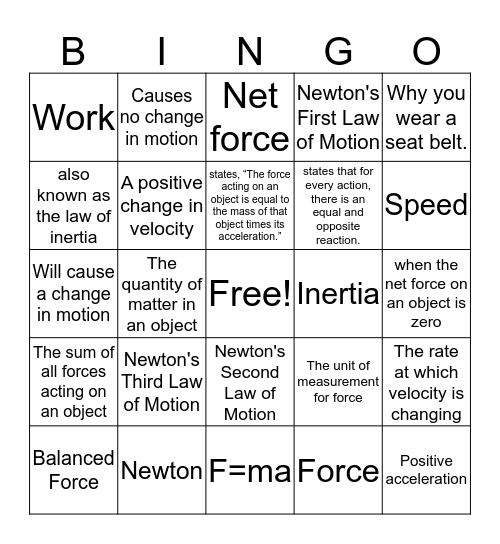# PHYSICS BINGOThis bingo card has a free space and 44 words: Acceleration, Positive acceleration, Negative acceleration, Balanced Force, Constant Speed, Force, Net force, Unbalance Force, Why you wear a seat belt., Newton, Newton's First Law of Motion, Inertia, Newton's Second Law of Motion, Newton's Third Law of Motion, Friction, F=ma, More force to move a rhino than a dog., Will cause a change in motion, Gravity, Speed, Causes no change in motion, Net Force = 42 N to the right, Velocity, Work, A push or a pull, when the net force on an object is zero, An object at rest remains at rest; and if in motion will remain in motion in a straight line; unless a force acts on it, This acts on a mass to create the force of weight, The unit of measurement for force, The sum of all forces acting on an object, An objects resistance to change its motion, The rate at which velocity is changing, Magnitude of distance traveled divided by time taken, speed + direction, The quantity of matter in an object, a change in position of an object, An objects resistance to change in its motion, A force that counteracts motion, states, “The force acting on an object is equal to the mass of that object times its acceleration.”, also known as the law of inertia, states that for every action, there is an equal and opposite reaction., A negative change in velocity, A positive change in velocity and The unit of measurement for forces.

## Play Online